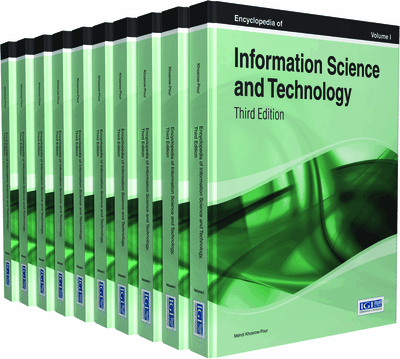# Reversible Logic as a Stepping Stone to Quantum Computing

J. E. Rice (University of Lethbridge, Canada)
DOI: 10.4018/978-1-4666-5888-2.ch715

Top

## Background

In 1961 Landauer defined a device as being logically irreversible if the output of the device did not uniquely define the inputs. He argued that logical irreversibility implied physical irreversibility, which unavoidably would lead to heat dissipation. However Landauer thought that the extra computation and/or memory required to achieve reversibility would offset the inherent advantages (Landauer, 1961). Bennett refuted this in 1973 and demonstrated that it was possible to design a reversible equivalent that was not significantly more complex than an equivalent irreversible device. Bennett also demonstrated that for power not to be dissipated it is necessary that a binary circuit be constructed from reversible gates (Bennett, 1973).

Following in Landauer and Bennett’s footsteps, Toffoli wrote that “...it appears possible to design circuits whose internal power dissipation, under ideal physical circumstances, is zero”. Toffoli also demonstrated that it is always possible to construct an arbitrary function by means of an invertible function (Toffoli, 1980). The current literature in reversible logic can almost entirely be traced back to these papers.

### Basic Terminology

In this article all operators are Boolean operators and standard symbols are used. While it is common practice to refer to “inputs” and “outputs” of a reversible function, this is not technically correct. A reversible function may be implemented using some quantum technology where wires and traditional technologies cannot be used and where inputs/outputs are really starting/ending states of some technological entity implementing the function. A more accurate term might be “qubits”, which is used by some authors; however many refer instead to lines, variables, or inputs and outputs of the circuit, assuming that these will be implemented by some reversible technology equivalent.

## Key Terms in this Chapter

Quantum Computing: An area of study focused on developing computer techniques and technologies based on the principles of quantum theory.

Reversible Logic: Either a) an area of study focusing on the design of logic circuits that implement bijective functions, or b) a logic function and/or circuit that implements a function that is bijective.

Circuit Design: An area of study focusing on the creation of circuits such as are used in computational devices; goals of this area are to achieve optimal sized circuits, circuits with minimal delay, and in the case of reversible computing, circuits with the lowest quantum cost and numbers of garbage lines.

Reversible Gate: A gate such as is used in the design of logic/computational circuits, but with the special property that the function it implements is bijective.

Reversible Computing: A model of computing in which the computational process to be carried out is either of itself reversible; that is, the inputs can be uniquely determined from the resulting outputs, or the underlying platform for the computational process is reversible.

## Complete Chapter List

Search this Book:
Reset